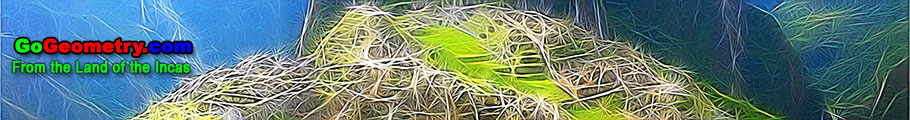Pentagons, Theorems and Problems - Table of ContentEuclid's Elements Book I, 23 Definitions. One-page visual illustration. Euclid's Elements Book. Index Word Cloud of Pentagon. Visual Summary. Euclid's Elements, Book XIII, Proposition 10 Pentagon, Hexagon, and Decagon. One page visual illustration.Proposition 15. Circle and regular pentagon. Miquel's Pentagram Theorem Interactive proof with animation and key theorems. Miquel's Pentagram with Dynamic Geometry. You can alter the pentagram dynamically in order to test and prove (or disproved) conjectures and gain mathematical insight that is less readily available with static drawings by hand. Requires Java Plug-in 1.3 or higher. Please be patient while the applet loads on your computer. Optical Prism with Regular Pentagonal Form. Scene created using Prism HD for iPad. iPad Apps: fractBG. Fractal Image with Pentagons. Dynamic Geometry 1477. Miquel's Pentagram Theorem, Pentagon, Triangle, Circumcircles, Concyclic Points, Step-by-step Illustration. GeoGebra, iPad. Geometry Problem 1141. Inscribed Pentagon, Circle, Triangle, Congruence, Midpoint, Concurrency. Geometry Problem 771 Area of a Parallelogram, Star, Pentagon, Triangles. Proposed Problem 430. Circumscribed and Inscribed Regular Pentagon, Perpendicular, Areas. Proposed Problem 429. Circumscribed and Inscribed Regular Pentagon, Perpendicular, Measurement. Proposed Problem 410. Two Regular Pentagons, Angle. Proposed Problem 342. Pentagon, Inscribed circle, Tangent, Semiperimeter. Proposed Problem 261. Regular Pentagon inscribed in a circle, sum of distances. Experiencing Geometry Poem. Taken from "Experiencing Geometry on Plane and Sphere," a classic textbook on Euclidean and non-Euclidean space. Used by permission of the author David W. Henderson. Pentagons illustration. Proposed Problem 169. Parallelogram, Interior and Exterior Points, Diagonals, Pentagon, Triangles, Areas. Proposed Problem 168. Parallelogram, Diagonal, Triangles, Pentagon, Areas. Proposed Problem 225. Viviani's Theorem Extension, Regular Polygon, Apothem, Distance. Interactive Mind Map of the Van Hiele Model of Geometric Thought Level 0. (Basic Level) Visualization. Index, Platonic Solids, Interactive animation, Tetrahedron in the cube, Archimedes and the Rhombicuboctahedron with animation, In the News, Pentagons & Pentagrams. I found, exploring with dynamic geometry software, new facts about pentagons and pentagrams. Key concept: Menelaus Theorem.

 Home | Search | Geometry | Polygon | Post a comment |Email | By Antonio Gutierrez Last updated: May 1, 2020